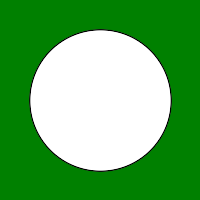# Wand circle() function in Python

• Last Updated : 11 May, 2020

The circle() function is another Drawing function in Wand. This method is used to draw a circle in the image. It requires only two arguments that are origin and perimeter of the circle.

Syntax: wand.drawing.circle(origin, perimeter)

Attention geek! Strengthen your foundations with the Python Programming Foundation Course and learn the basics.

To begin with, your interview preparations Enhance your Data Structures concepts with the Python DS Course. And to begin with your Machine Learning Journey, join the Machine Learning - Basic Level Course

Parameters :

ParameterInput TypeDescription
origin(collections.abc.Sequence)or(Real, numbers.Real)pair which represents origin x and y of circle.
perimeter(collections.abc.Sequence)or(Real, numbers.Real)pair which represents perimeter x and y of circle

Example #1:

 `# Import required objects from wand modules``from` `wand.image ``import` `Image``from` `wand.drawing ``import` `Drawing``from` `wand.color ``import` `Color`` ` `# generate object for wand.drawing``with Drawing() as draw:`` ` `    ``# set stroke color``    ``draw.stroke_color ``=` `Color(``'black'``)`` ` `    ``# set width for stroke``    ``draw.stroke_width ``=` `1`` ` `    ``# fill white color in arc``    ``draw.fill_color ``=` `Color(``'white'``)``    ``origin ``=` `(``100``, ``100``)``    ``perimeter ``=` `(``50``, ``50``)`` ` `    ``# draw circle using circle() function``    ``draw.circle(origin, perimeter) ``    ``with Image(width ``=` `200``,``               ``height ``=` `200``,``               ``background ``=` `Color(``'green'``)) as img:`` ` `        ``# draw shape on image using draw() function``        ``draw.draw(img)``        ``img.save(filename ``=``'circle.png'``)`

Output:Example #2:
Input Image:`# Import required objects from wand modules``from` `wand.image ``import` `Image``from` `wand.drawing ``import` `Drawing``from` `wand.color ``import` `Color`` ` `# generate object for wand.drawing``with Drawing() as draw:``    ``origin ``=` `(``100``, ``100``)``    ``perimeter ``=` `(``50``, ``50``)`` ` `    ``# set stroke color``    ``draw.stroke_color ``=` `Color(``'black'``)`` ` `    ``# set width for stroke``    ``draw.stroke_width ``=` `1`` ` `    ``# fill white color in arc``    ``draw.fill_color ``=` `Color(``'white'``)`` ` `    ``# draw bezier curve using bezier function``    ``draw.circle(origin, perimeter)``    ``with Image(filename ``=``"gog.png"``) as img:`` ` `        ``# draw shape on image using draw() function``        ``draw.draw(img)``        ``img.save(filename ``=``'circle2.png'``)`

Output:My Personal Notes arrow_drop_up